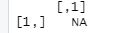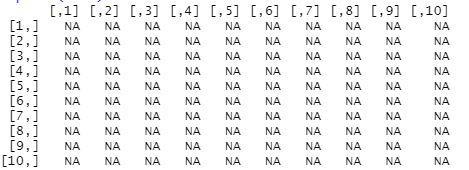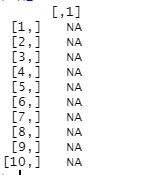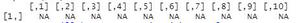Related Articles

# How to create an empty matrix in R ?

• Last Updated : 24 Sep, 2021

The term empty matrix has no rows and no columns. A matrix that contains missing values has at least one row and column, as does a matrix that contains zeros. In this article, we are going to see how to create an empty matrix in R Programming Language.

There are three ways of creating an empty matrix:

• Using row and column.
• Using only row.
• Using only column.

Method 1: Using both row and column:

Here in this, we need to pass both row and column to create an empty matrix:

Syntax: matrix name = matrix(, nrow = value 1, ncol = value2)

Where:

• Here matrix name can be any valid identifier
• Value 1 is for number of rows.
• Value 2 is for number of columns.

Example 1: In the below example, we created a mat variable, After creating mat variable we are using the matrix function to create a matrix and mentioning a number of rows and columns in it.

Below is the implementation:

## R

 `# creating empty matrix,``# storing in variable mat and passing``# number of rows and columns``mat = ``matrix``(, nrow = 1, ncol = 1)` `# printing empty matrix.``print``(m)`

Output:Here we got NA as output which means not a number or not available.

Example 2:

## R

 `# creating empty matrix,``# storing in variable mat1 and passing``@ number of rows and columns``mat1 = ``matrix``(, nrow = 10, ncol = 10)` `# printing empty matrix.``print``(mat1)`

Output:Method 2: Using only row :

Here we need to pass the only one row to create an empty matrix

Syntax: matrix name = matrix(, nrow = value 1)

Where,

Here matrix name can be any valid identifier

value 1 is for number of rows.

Example 1:

## R

 `Mat<-``matrix``(,nrow=10)` `# printing empty matrix.``print``(Mat)`

Output:Method 3: Using only column.

Here we need to pass the only columns to create an empty matrix.

Syntax: matrix name = matrix(, ncol = value 1)

Where,

Here matrix name can be any valid identifier

Value 1 is for number of column.

Below is the implementation:

## R

 `Mat<-``matrix``(,ncol=10)` `#printing empty matrix.``print``(Mat)`

Output:My Personal Notes arrow_drop_up